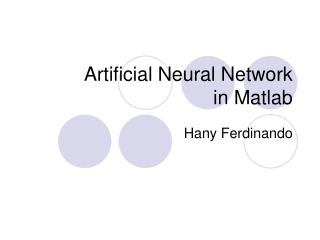DownloadDownload PresentationArtificial Neural Network in Matlab

# Artificial Neural Network in Matlab

Télécharger la présentation## Artificial Neural Network in Matlab

- - - - - - - - - - - - - - - - - - - - - - - - - - - E N D - - - - - - - - - - - - - - - - - - - - - - - - - - -
##### Presentation Transcript

1. Artificial Neural Networkin Matlab Hany Ferdinando

2. Architecture (single neuron) w is weight matrices, dimension 1xR p is input vector, dimension Rx1 b is bias a = f(Wp + b) Neural Network in Matlab

3. Transfer Function Neural Network in Matlab

4. Architecture with neurons w is weight matrices, dimension SxR p is input vector, dimension Rxn b is bias Neural Network in Matlab

5. Multiple layers Neural Network in Matlab

6. Perceptrons in Matlab Make the perceptrons with net = newp(PR,S,TF,LF) PR = Rx2 matrix of min and max values for R input elements S = number of output vector TF = Transfer function, default = ‘hardlim’, other option = ‘hardlims’ LF = Learning function, default = ‘learnp’, other option = ‘learnpn’ hardlim = hardlimit function hardlims = symetric hardlimit function learnp Dw = (t-a)pT = epT learnpn  normalized learnp Wnew = Wold + DW bnew = bold + e where e = t - a Neural Network in Matlab

7. Compute manually… • This is an exercise how to run the artificial neural network • From the next problem, we will compute the weights and biases manually Neural Network in Matlab

8. AND Gate in Perceptron P = [0 0 1 1; 0 1 0 1]; T = [0 0 0 1]; net = newp([0 1; 0 1],1); weight_init = net.IW{1,1} bias_init = net.b{1} net.trainParam.epochs = 20; net = train(net,P,T); weight_final = net.IW{1,1} bias_final = net.b{1} simulation = sim(net,P) weight_init = [0 0], bias_init = 0 weight_final = [2 1], bias_final = -3 Neural Network in Matlab

9. OR Gate in Perceptron P = [0 0 1 1; 0 1 0 1]; T = [0 1 1 1]; net = newp([0 1; 0 1],1); weight_init = net.IW{1,1} bias_init = net.b{1} net.trainParam.epochs = 20; net = train(net,P,T); weight_final = net.IW{1,1} bias_final = net.b{1} simulation = sim(net,P) weight_init = [0 0], bias_init = 0 weight_final = [1 1], bias_final = -1 Neural Network in Matlab

10. NAND Gate in Perceptron P = [0 0 1 1; 0 1 0 1]; T = [1 1 1 0]; net = newp([0 1; 0 1],1); weight_init = net.IW{1,1} bias_init = net.b{1} net.trainParam.epochs = 20; net = train(net,P,T); weight_final = net.IW{1,1} bias_final = net.b{1} simulation = sim(net,P) weight_init = [0 0], bias_init = 0 weight_final = [-2 -1], bias_final = 2 Neural Network in Matlab

11. NOR Gate in Perceptron P = [0 0 1 1; 0 1 0 1]; T = [1 0 0 0]; net = newp([0 1; 0 1],1); weight_init = net.IW{1,1} bias_init = net.b{1} net.trainParam.epochs = 20; net = train(net,P,T); weight_final = net.IW{1,1} bias_final = net.b{1} simulation = sim(net,P) weight_init = [0 0], bias_init = 0 weight_final = [-1 -1], bias_final = 0 Neural Network in Matlab

12. Backpropagation in Matlab Make the backpropagation with net = newff(PR,[S1 S2...SNl],{TF1 TF2...TFNl},BTF,BLF,PF) PR = Rx2 matrix of min and max values for R input elements S = number of output vector BTF = Transfer function (user can use any transfer functions) BLF = Learning function PF = performance xk+1 = xk - akgk Neural Network in Matlab

13. Linear Filter (with ANN) in Matlab Make the Linear Filter with newlin(PR,S,ID,LR) PR = Rx2 matrix of min and max values for R input elements S = number of output vector ID = delay LR = Learning Rate Transfer function for linear filter is only linear line (purelin) Neural Network in Matlab